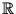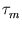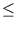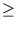The work was published in: Mat. Zametki 68 (2000), no. 4, pp. 483-503;

English translation: Math. Notes. 68 (2000), no. 4.

Arestov V.V. Babenko A.G.

Estimates of maximal value of angular code distance for 24 and 25 points on unit sphere in4

A B S T R A C T

This work devote to known problem about maximal cardinality(s) of spherical s-code (- 1s < 1) in m-dimensional Euclidean spacem, m2; more precisely, it is considered the Delsarte's function wm(s) which connected with(s) by inequality:(s)wm(s). Solution of the equation wm(s) = N for m = 4 and N = 24, 25 is found. As consequence, it is obtained that among arbitrary 25 (resp. 24) points located on unit sphere in4 there exist two points with the angular distance between them strictly less than 60.5o (resp. 61.41o).

Bibliogr. 24 titles.

Key words: spherical codes, kissing numbers, Chebyshev polynomials of the second kind.